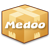version: 2.1.10

### avg

Calculate the average value of the column.

avg(\$table, \$column, \$where)
• ##### table [string]

The table name.

• ##### column [string]

The target column will be calculated.

• ##### where (optional) [array]

The WHERE clause to filter records.

avg(\$table, \$join, \$column, \$where)
• ##### table [string]

The table name.

• ##### join [array]

Table relativity for tables.

• ##### column [string]

The target column will be calculated.

• ##### where (optional) [array]

The WHERE clause to filter records.

Return: [string] The average number of the column.
```\$average = \$database->avg("account", "age", [
"gender" => "male"
]);

echo "The average age of the male user is " . \$average;```